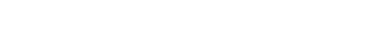#### Revision Notes

##### Aren’t histograms just really hard bar charts?

No! There are many mathematical differences that you should be aware of but the key difference between a bar chart and a histogram is that with a histogram it is the area of the bars that determine the frequency, on a bar chart it’s the height (or length) of them.

Digging deeper, histograms are used for continuous data (bar charts for discrete) and are particularly useful when data has been grouped into different sized classes.

But the key thing to getting started is that it is the area of the bars that tell us what is happening with the data.  This means, unlike any other graph or chart you have come across, it is very difficult to tell anything from simply looking at a histogram, you have to drill down into the numbers and detail.

##### What do I need to know?

You need to know how to draw a histogram (most questions will get you to finish an incomplete histogram).

When drawing histograms we will need to use frequency density (fd):You’ll also need to be able to work backwards from a given histogram to find frequencies and estimate the mean.

1. Drawing a histogram

From a given table you need to work out the frequency density for each class.

Then you can plot the data against frequency density with frequency density on the y-axis.

eg Plot a histogram for the following data regarding the average speed travelled by trains.The class width column isn’t essential but it is crucial you show the frequency densities.

Now we draw bars (touching, as the data (speed) is continuous) with widths of the class intervals and heights of the frequency densities.

2. Interpreting Histograms

We shall still use the example above here but shall pretend we never had the table of data and were only given the finished histogram.

(a) To estimate the mean:

You need to know the total frequency and what all the data values add up to.  You can’t find the exact total of the data values as this is grouped data but we can estimate it using midpoints.

Since frequency density = frequency ÷ class width then it is easy to rearrange to see that frequency = frequency density × class width

20≤s<40: frequency = 0.25 x 20 = 5   midpoint = 30

40≤s<50: frequency = 1.5 x 10 = 15    midpoint = 45

50≤s<55: frequency = 5.6 x 5 = 28     midpoint = 52.5

55≤s<60: frequency = 7.6 x 5 = 38     midpoint = 57.5

60≤s<70: frequency = 1.4 x 10 = 14    midpoint = 65

Total of frequencies = 5 + 15 + 28 + 38 + 14 = 100

You can draw all of the above in a table if you wish

Now you can total up (an estimate of) the data values and find the mean:

Total = 5 x 30 + 15 x 45 + 28 x 52.5 + 38 x 57.5 + 14 x 65 = 5390

(Be careful if you type all this into your calculator in one go!)

Estimate of Mean = 5390 ÷ 100 = 53.9

#### Example(b)#### Question#### Answer## Want to aim for a Level 9?

See if you’ve got what it takes. Test yourself with our topic questions.### Author: Simon

After 24 years teaching A Level Further Maths, Maths whizz Simon focused his attention on tutoring. He’s helped thousands of young people make sense of Maths and now he’s helping you too – aren’t you lucky! He’s even worked as an Edexcel examiner, so knows exactly what they’re looking for.